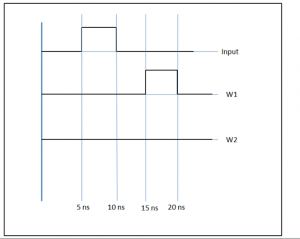Q.1
##### Which of the following is default delay in VHDL?
• a) Inertial delay
• b) Transport delay
• c) Delta delay
• d) Wire delay
Q.2
• a) Time
• b) Error
• c) Inertia
• d) Pulse
Q.3
##### The inertia value in inertial delay model is equal to _________
• a) Initial value
• b) Delay
• c) Input value at a specific time
• d) Output value at a specific time
Q.4
##### Transport delay is a kind of __________
• a) Synthesis delay
• b) Simulation delay
• c) Inertial delay
• d) Wire delay
Q.5
• a) True
• b) False
Q.6
##### A buffer with single input A and single output B has a delay ofnanosecond. If the value of input A changes afterns from 0 to 1 and it changes again from 1 to 0 atns. At what time, the value of output B will beif the inertial delay model is used?
• a) 30 ns
• b) 40 ns
• c) 20 ns
• d) Output will remain zero
Q.7
##### The keyword TRANSPORT in any assignment statement specifies _______
• a) Transport delay
• b) Transfer the right operand immediately to left operand
• c) Transporting the value of left operand to right operand
• d) Inertial delay
Q.8
##### A buffer with single input A and single output B has a delay ofnanosecond. If the value of input A changes afterns from 0 to 1 and it changes again from 1 to 0 atns. At what time, the value of output B will beif the transport delay model is used?
• a) 20 ns
• b) 30 ns
• c) 40 ns
• d) Output will remain zero
Q.9
##### In the VHDL code given below, which delay model is used? LIBRARY IEEE; USE IEEE.std_logic_1164.all; ENTITY buffer IS PORT(a : IN STD_LOGIC; b : OUT STD_LOGIC); END buffer; ARCHITECTURE buf OF buffer IS BEGIN b <= a AFTERns; END buf;
• a) Simulation delta model
• b) Transport delay model
• c) Inertial delay model
• d) Multiple driver delay model
Q.10
##### Following waveform shows the output B of a buffer having delayns for two different delay mechanisms, specify the name of delay mechanism for corresponding waveform.
• a) W1- Inertial, W2- Transport
• b) W1- Inertial, W2- Inertial
• c) W1- Transport, W2- Transport
• d) W1- Transport, W2- Inertial
Q.11
##### For zero delay events, which of the following mechanism is used?• a) Transport delay mechanism
• b) Inertial delay mechanism
• c) Delta delay mechanism
• d) Preemption delay mechanism
Q.12
##### Which of the following delay model follows the principle of preemption?
• a) Inertial delay
• b) Transport delay
• c) Delta delay
• d) Wire delay
Q.13
##### Which of the following is not the application of inertial delay?
• a) Buffer delay
• b) PC wire line delay
• c) Simple delay in OR gate
• d) Inverter delay
Q.14
##### The condition to implement the simulation delta delay is _______
• a) All events must be synchronous
• b) The events must have at least one sequential circuit
• c) No condition
• d) All events must be zero delay event